# 8. [10 points) Consider the following algorithm procedure Algorithm(: integer, n: positive integer; 81,...a s integers with vhilei<r print (l, r, mı, arn, 》 if z > am then 1:= m + 1 if za...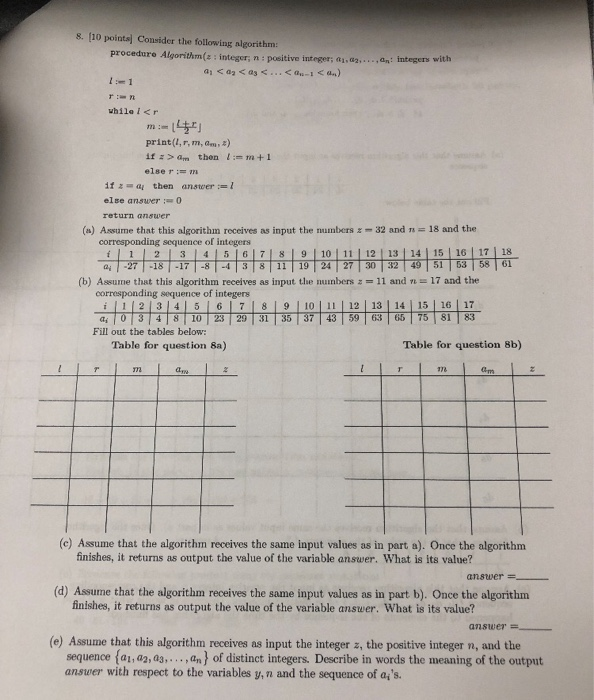Question 8 a):

 1 18 9 19 32 10 18 14 49 32 10 14 12 30 32 13 14 13 32 32

Question 8 b):

 1 17 9 35 11 1 9 5 10 11 6 9 7 29 11 6 7 6 23 11

Question 8 c):

Question 8 d):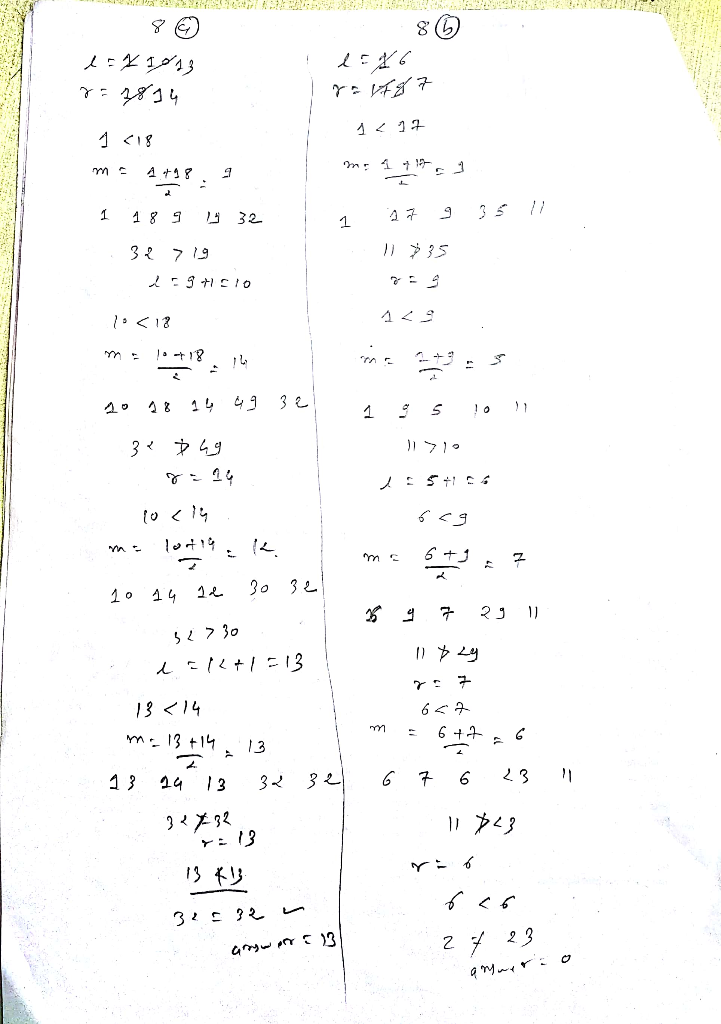#### Earn Coin

Coins can be redeemed for fabulous gifts.

Similar Homework Help Questions
• ### Consider the following algorithm: ocedure Algorithm (b: integer, n: positive integer,i datinct integem) proc answer :", 0 nand 6 while (j print(j, z, b, answer) if jSn then answer:-j return a...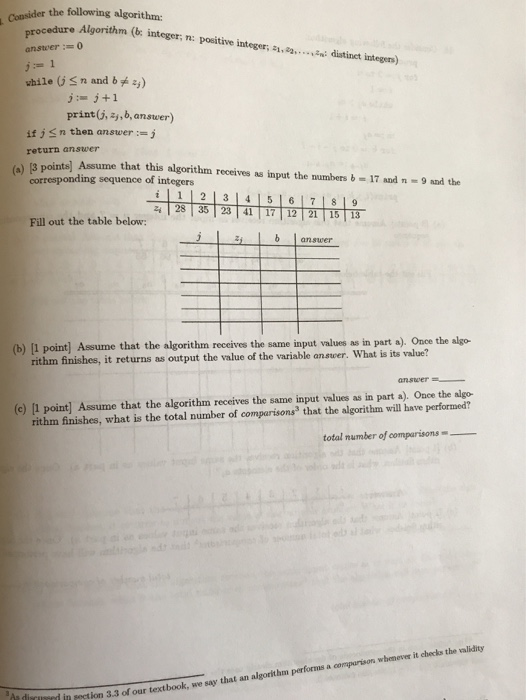Consider the following algorithm: ocedure Algorithm (b: integer, n: positive integer,i datinct integem) proc answer :", 0 nand 6 while (j print(j, z, b, answer) if jSn then answer:-j return answer (8 points] Assume that this algorithm receives as input the numbers b-17 andn9nd the corresponding sequence or iaie i 2 3 4 516 7 8 corresponding sequence of integers 19 Fill out the table below: i 는, ↓answer (b) [I point] Assume that the algorithm receives the same input...

• ### 9. [10 points) Consider the following algorithm: procedure Algorithm(n: positive integer; ddd: distinet integers) for k:=1 to n-1 for 1-1 to n-k print(k, I, di,da...-1,dn) if ds dti then interch...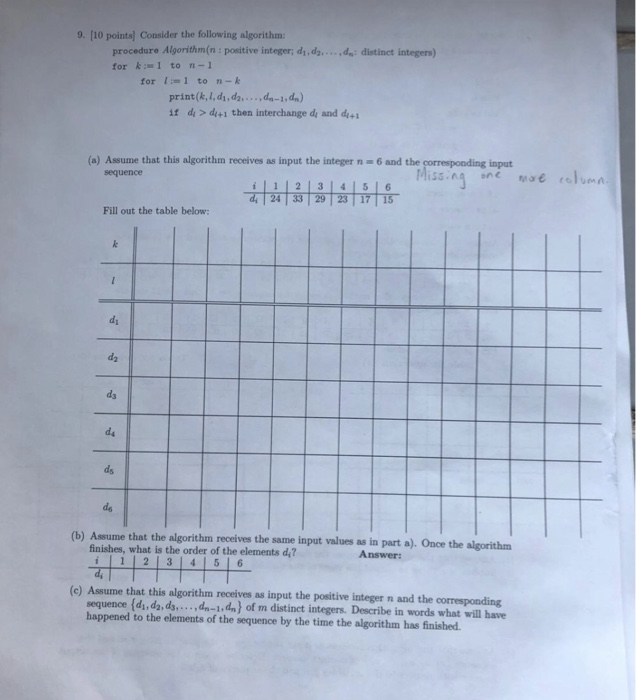9. [10 points) Consider the following algorithm: procedure Algorithm(n: positive integer; ddd: distinet integers) for k:=1 to n-1 for 1-1 to n-k print(k, I, di,da...-1,dn) if ds dti then interchange dy and d (a) Assume that this algorithm receives as input the integer n 6 and the input sequence 하하하하하하, Miss ^-ruteae rehen i12|3141516 Fill out the table below: ds ds (b) Assume that the algorithm receives the same input values as in part a). Once the algorithm finishes, what...

• ### 17. Consider the following algorithm: procedure Algorithm(n: positive integer; di,d2.. ,dn: distinct integers) for 1 to n-1 for 1 to n-k if ddi+ then interchange di and di+ print(k, I, d,ddn-1, d...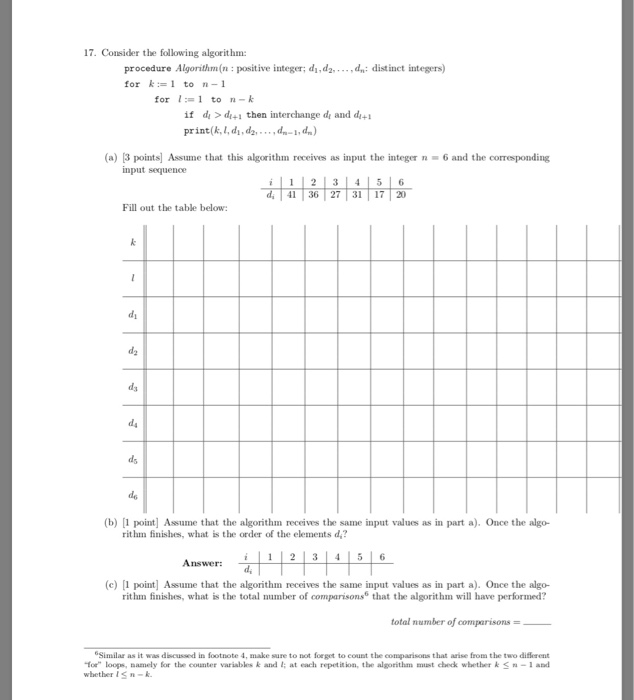17. Consider the following algorithm: procedure Algorithm(n: positive integer; di,d2.. ,dn: distinct integers) for 1 to n-1 for 1 to n-k if ddi+ then interchange di and di+ print(k, I, d,ddn-1, dn) (a) |3 points Assume that this algorithm receives as input the integer-6 and the corresponding input sequence 41 36 27 31 17 20 Fill out the table below ds (b) 1 point Assume that the algorithm receives the same input values as in part a). Once the algo-...

• ### 2. Let d, n be integers with d关0, We say "d divides n" (denote d|n) if there exists an integer r such that m-d r. In this case, we also say that d is a divisor of n. A prime is a positive in...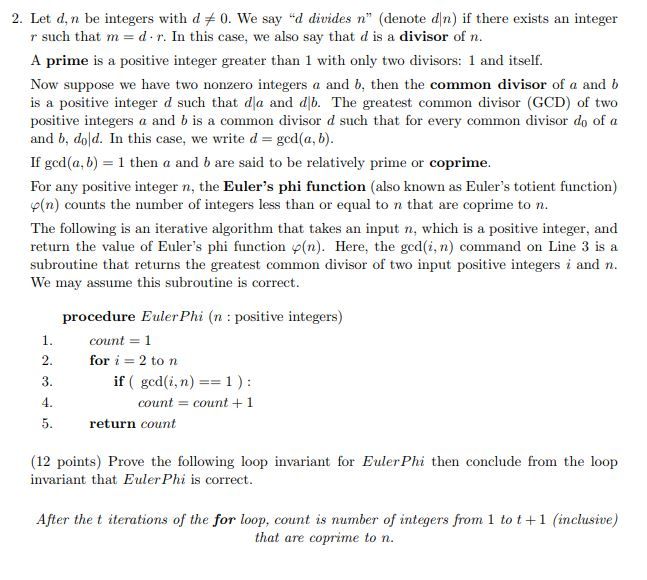2. Let d, n be integers with d关0, We say "d divides n" (denote d|n) if there exists an integer r such that m-d r. In this case, we also say that d is a divisor of n. A prime is a positive integer greater than 1 with only two divisors: 1 and itself. Now suppose we have two nonzero integers a and b, then the common divisor of a and b is a positive integer d such that dla...

• ### 1 1 point Consider the following algorithm for factoring an integer N provided as input (in...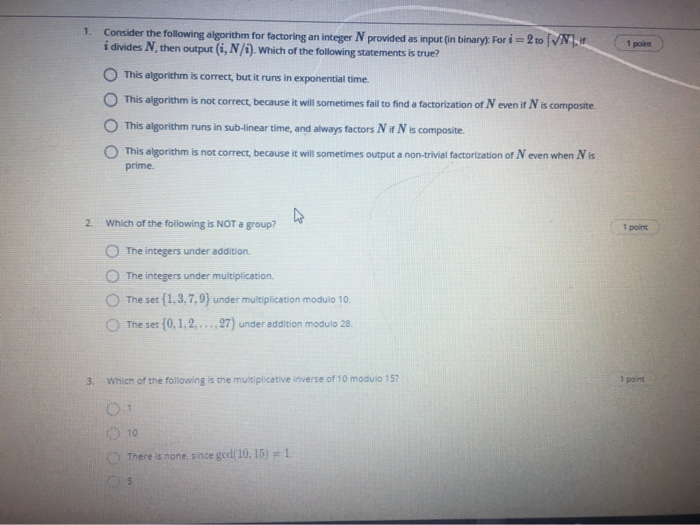1 1 point Consider the following algorithm for factoring an integer N provided as input (in binary): For i = 2 to [VN.17 i divides N, then output (i, N/). Which of the following statements is true? This algorithm is correct, but it runs in exponential time. This algorithm is not correct, because it will sometimes fail to find a factorization of Neven if N is composite This algorithm runs in sub-linear time, and always factors N it Nis composite...

• ### 20. [5 points] Rewrite the following ML. function using patterns fun factn. ifn-0 then 1 else n fact (n-1) 21. [S points) Using map function, write an ML function int2real of type int list real l...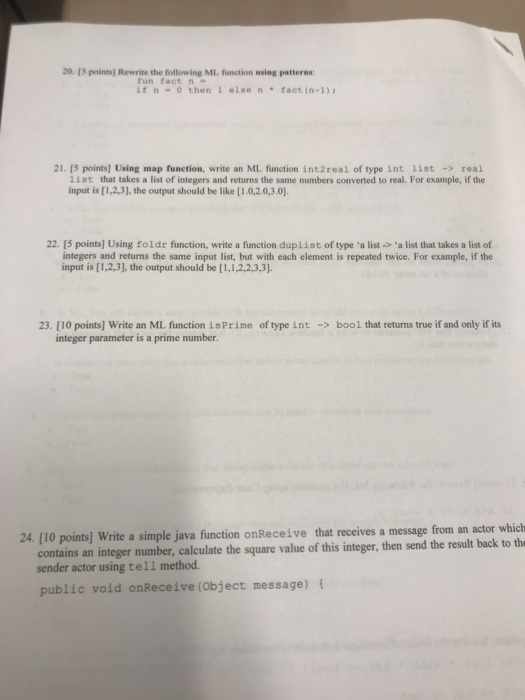20. [5 points] Rewrite the following ML. function using patterns fun factn. ifn-0 then 1 else n fact (n-1) 21. [S points) Using map function, write an ML function int2real of type int list real list that takes a list of integers and returns the same numbers converted to real. For example, if the input is [1,2,3], the output should be like [1.0,2.0,3.0 22. [5 points] Using foldr function, write a function duplist of type·a list 'a list that takes...

• ### COMPUTER SCIENCE (URGENT)

DO WHAT YOU CAN DO FOR NOW , YOU CAN OTHER QUESTIONS LATER . IT'S DUE IN 2 WEEKS FROM TOMORROW WORTH 100POINT.PSS: IF YOU DON'T KNOW HOW TO DO IT PLEASE LEAVE IT BLANKPSS:IF YOU ARE MR "IT'S ME" AGAIN PLEASE LET ME KNOW"1.A function that is called or summoned into action by its reference in another function is a ____.A) function prototypeB) called functionC) calling functionD) function declarator2.The items enclosed within the parentheses in a function call statement...